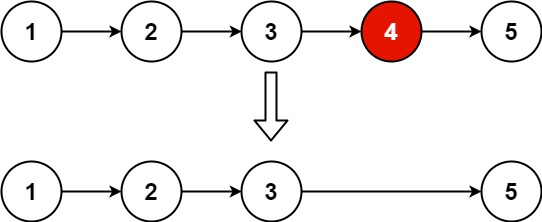# GeetCode Hub

Given the `head` of a linked list, remove the `nth` node from the end of the list and return its head.

Example 1:```Input: head = [1,2,3,4,5], n = 2
Output: [1,2,3,5]
```

Example 2:

```Input: head = , n = 1
Output: []
```

Example 3:

```Input: head = [1,2], n = 1
Output: 
```

Constraints:

• The number of nodes in the list is `sz`.
• `1 <= sz <= 30`
• `0 <= Node.val <= 100`
• `1 <= n <= sz`

Follow up: Could you do this in one pass?

/** * Definition for singly-linked list. * public class ListNode { * int val; * ListNode next; * ListNode() {} * ListNode(int val) { this.val = val; } * ListNode(int val, ListNode next) { this.val = val; this.next = next; } * } */ class Solution { public ListNode removeNthFromEnd(ListNode head, int n) { if(head == null){ return null; } ListNode dummy = new ListNode(0); dummy.next = head; ListNode slow = dummy; ListNode fast = dummy; for(int i=1 ;i<= n+1;i++){ fast = fast.next; } while(fast!=null){ slow = slow.next; fast = fast.next; } slow.next = slow.next.next; return dummy.next; } }

The main concept to remember in this question is how we can use two pointers, i.e slow and fast pointers, to find the node that needs to be deleted. Once that is found then it is an easy task to delete the node.

Time Complexity: O(n)

Space Complexity: O(1)